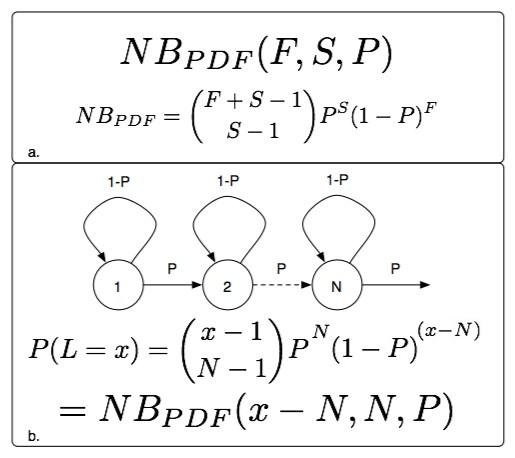## November 12, 2004

### The Negative Binomial Distribution meets the State MachineThe negative binomial distribution captures the probability of having S-1 successes and F failures in F+S-1 trials and then a success on the (F+S)th trial where the probability of success at any trial is P. Let this function be called NBpdf(F,S,P). If a N-state, left-right markov model is constructed such that the probability of transition to the next state is P and the probability of a self-transition is (1-P), then the probability of visiting x states (and generating an observation sequence of length x) in a state machine of length n is given by NBpdf(x-N,N,P).
Posted by djp3 at 8:01 AM | Comments (0) | TrackBack (0)

## November 11, 2004

### Continuous versus Discrete Distributions

Jeff Bilmes makes an excellent point about distributions and talking about them correctly. In particular:

An exponential distribution is a continous distribution.
A geometric distribution is the discrete version.

A gamma distribution is a continuous distribution.
A negative binomial distribution is the the discrete analog.
Posted by djp3 at 5:08 PM | Comments (0) | TrackBack (0)